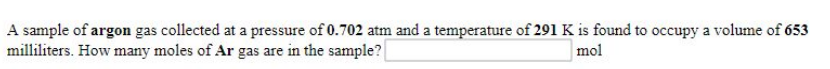# Problem: A sample of argon gas collected at a pressure of 0.702 atm and a temperature of 291 K is found to occupy a volume of 653 millimeters. How many moles of Ar gas are in the sample?

###### FREE Expert Solution
100% (484 ratings)###### Problem Details

A sample of argon gas collected at a pressure of 0.702 atm and a temperature of 291 K is found to occupy a volume of 653 millimeters. How many moles of Ar gas are in the sample?